# Fibonacci coding

Known as: Fibonacci code, Fibonacci encoding, Fibonacci representation
In mathematics and computing, Fibonacci coding is a universal code which encodes positive integers into binary code words. It is one example of… Expand
Wikipedia

## Papers overview

Semantic Scholar uses AI to extract papers important to this topic.
2014
2014
• ArXiv
• 2014
We implement a decision procedure for answering questions about a class of infinite words that might be called (for lack of a… Expand
•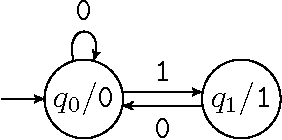•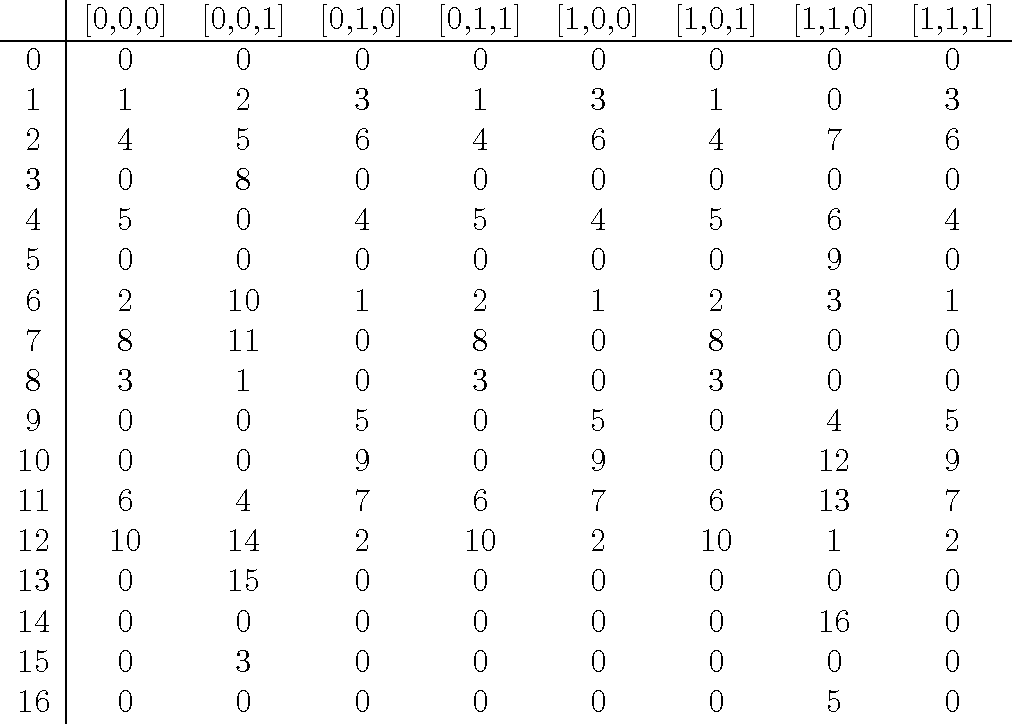•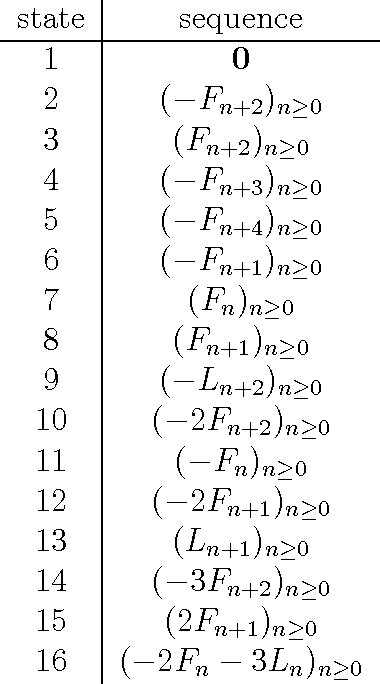•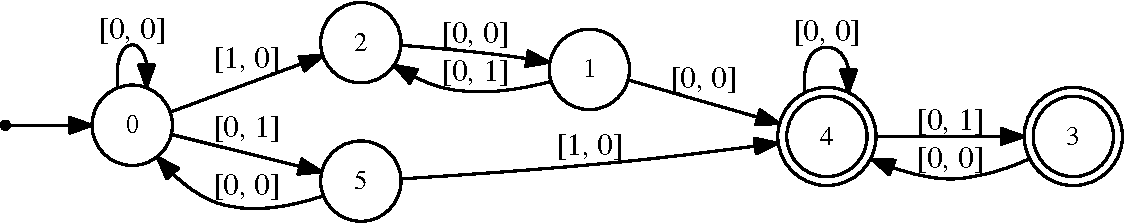•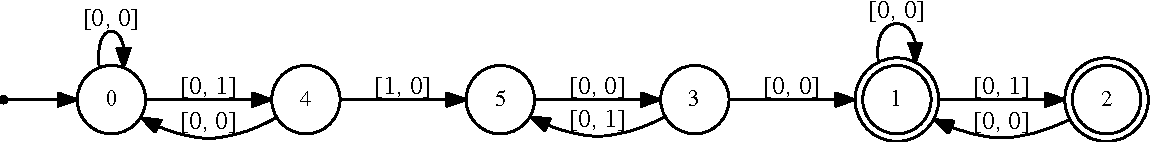Is this relevant?
2013
2013
David S. Simon,1,2 Nate Lawrence,2 Jacob Trevino,3 Luca Dal Negro,2,3,* and Alexander V. Sergienko2,4,† 1Department of Physics… Expand
•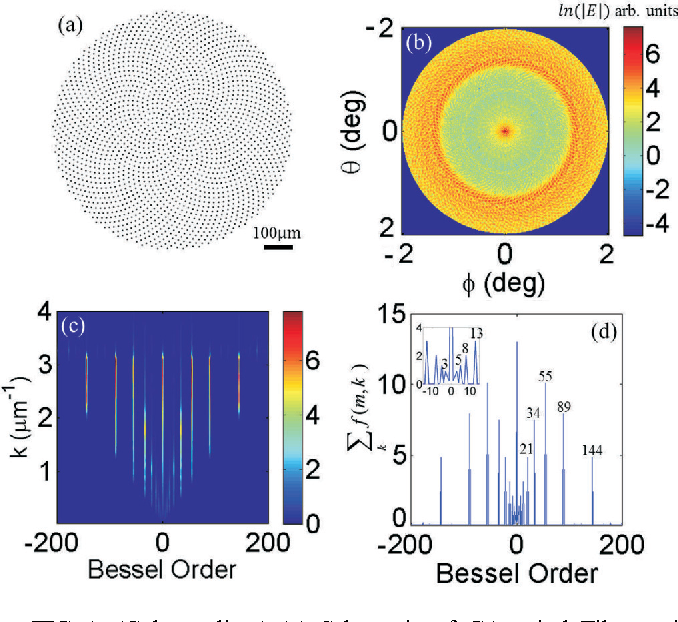•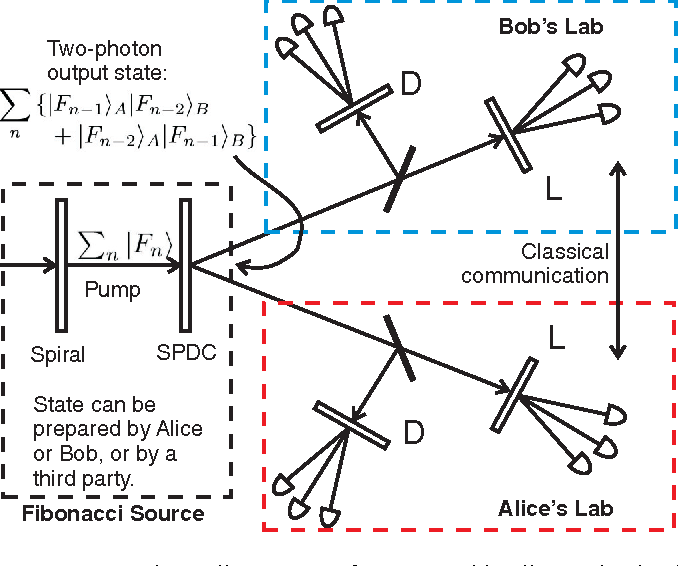•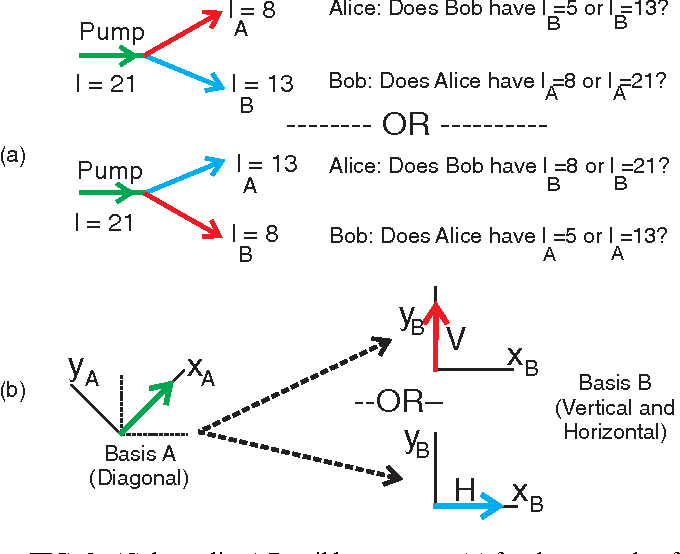•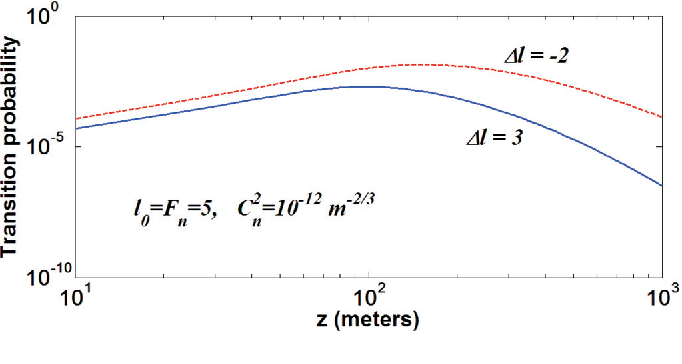•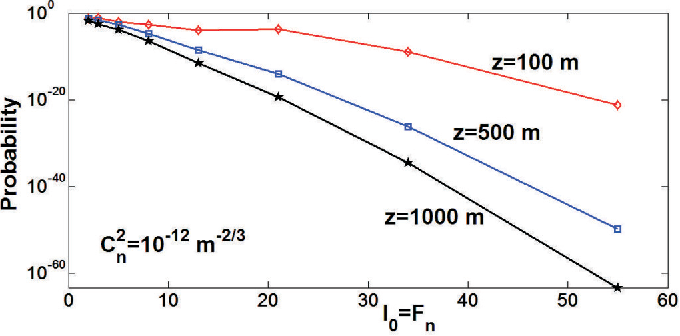Is this relevant?
2012
2012
• Eur. J. Comb.
• 2012
For a binary word f, let Q"d(f) be the subgraph of the d-dimensional cube Q"d induced on the set of all words that do not contain… Expand
•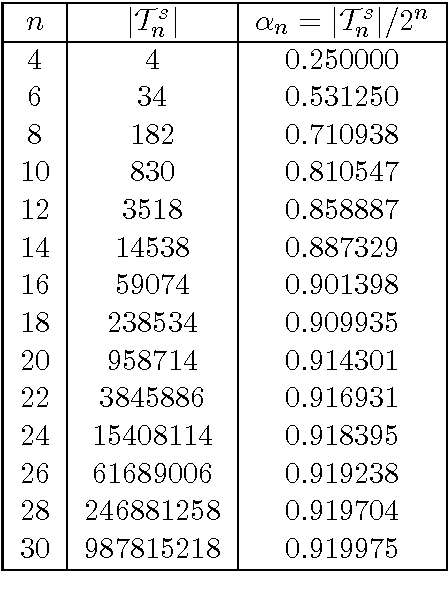•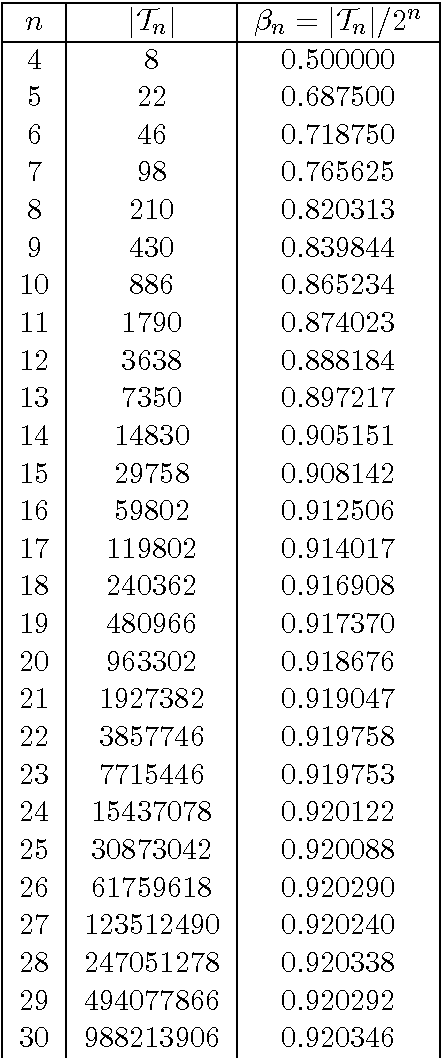Is this relevant?
2010
2010
Let $\varphi=(1+\sqrt{5})/2$ denote the golden section. We investigate relationships between unbounded iterations of the floor… Expand
•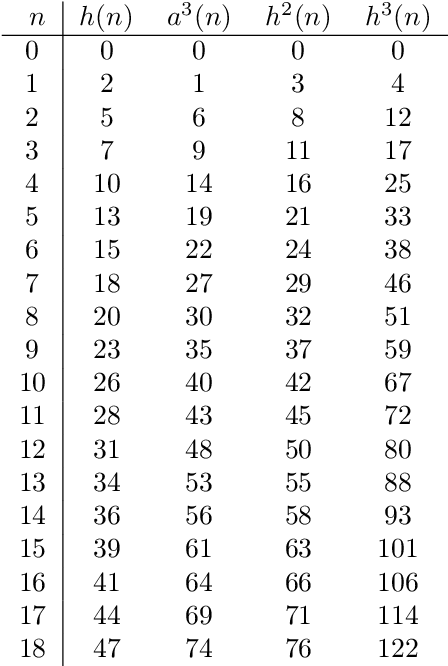•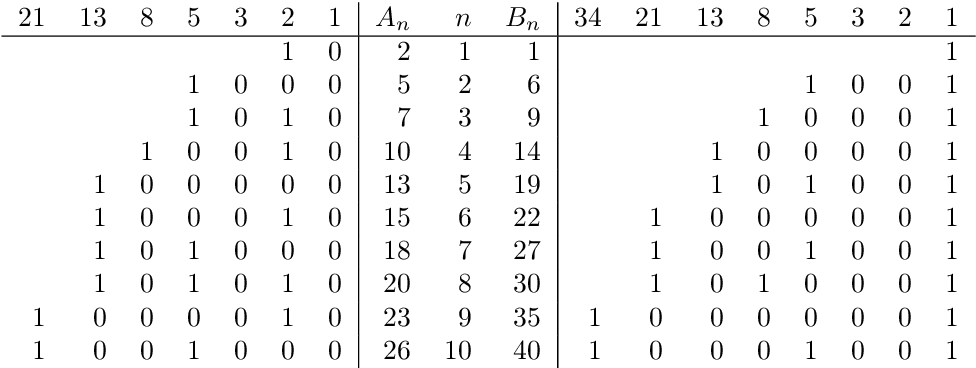•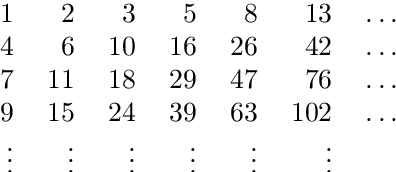•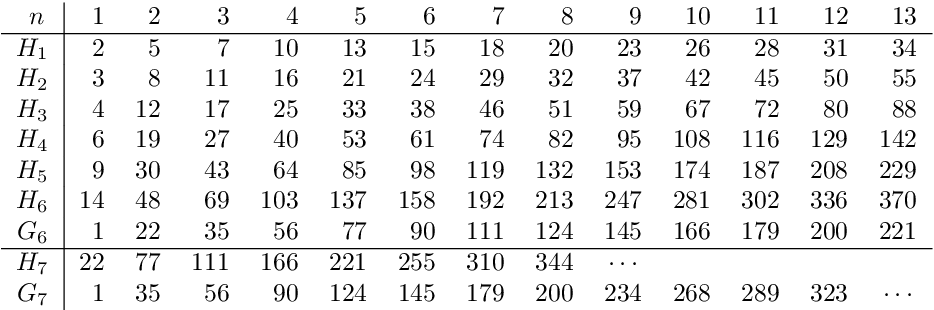Is this relevant?
2010
2010
Burrows-Wheeler data compression algorithm (BWA) is one of the most effective textual data compressors. BWA includes three main… Expand
•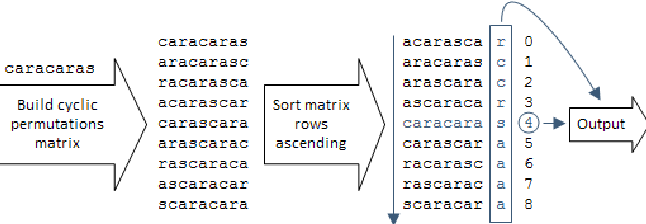•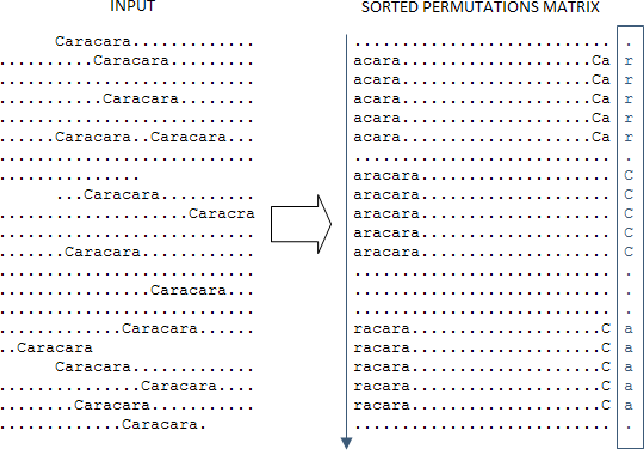•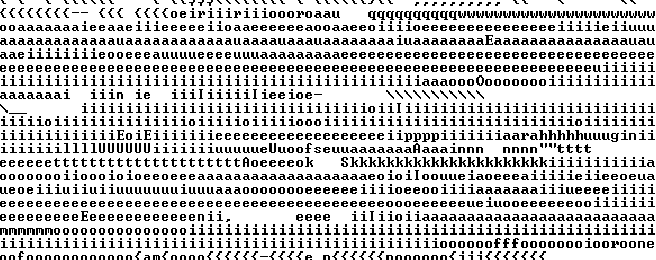•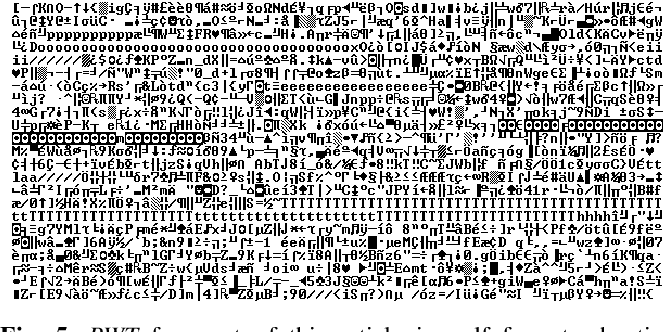•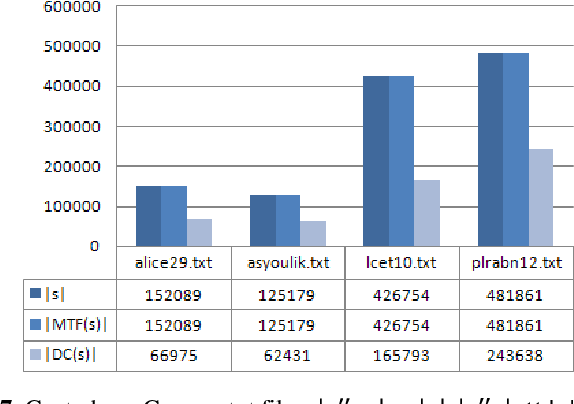Is this relevant?
2009
2009
The numerical semigroup generated by relatively prime positive integers a1, . . . , an is the set S of all linear combinations of… Expand
Is this relevant?
2007
2007
• Algorithmica
• 2007
Abstract Fibonacci cubes are induced subgraphs of hypercubes based on Fibonacci strings. They were introduced to represent… Expand
•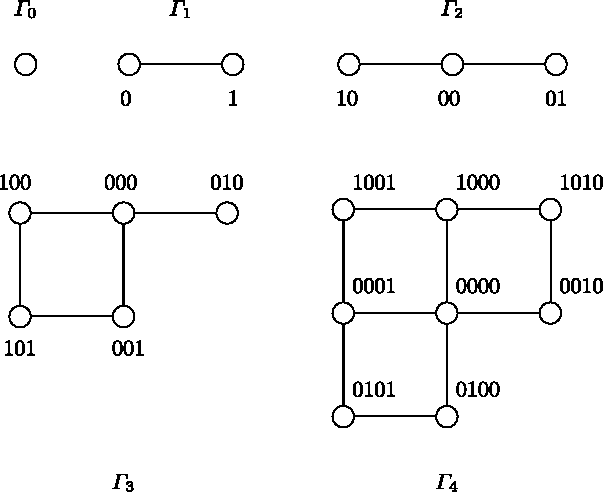•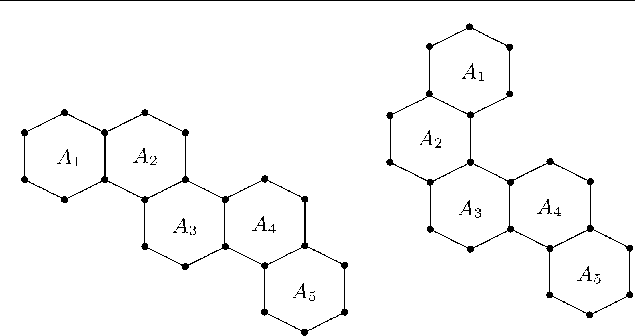•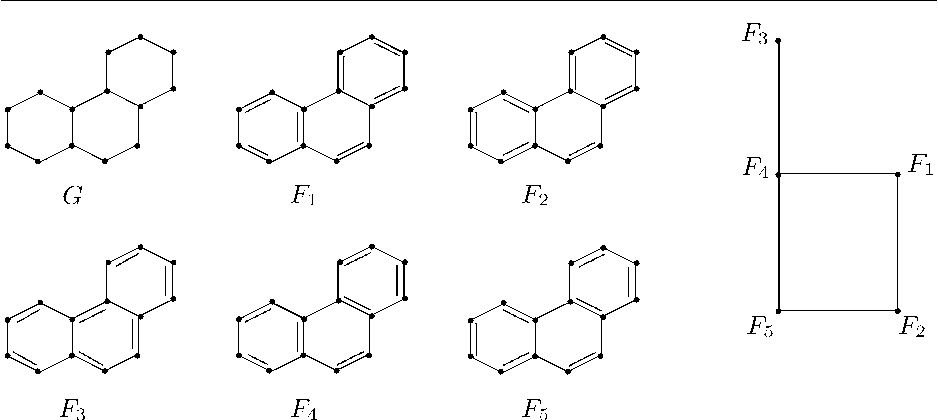•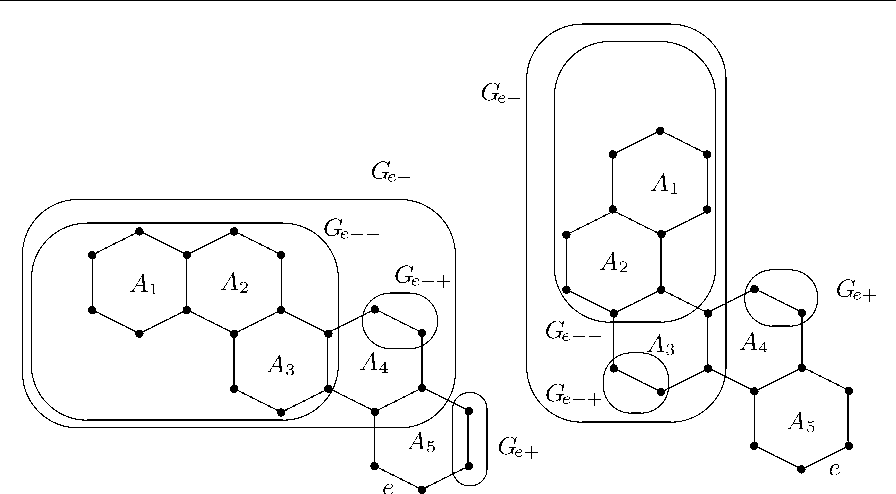•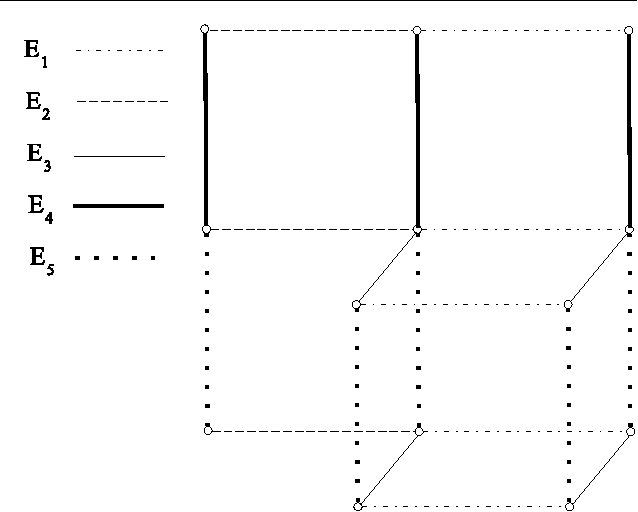Is this relevant?
2006
2006
Abstract We consider a new class of square Fibonacci ( p  + 1) × ( p  + 1)-matrices, which are based on the Fibonacci p -numbers… Expand
•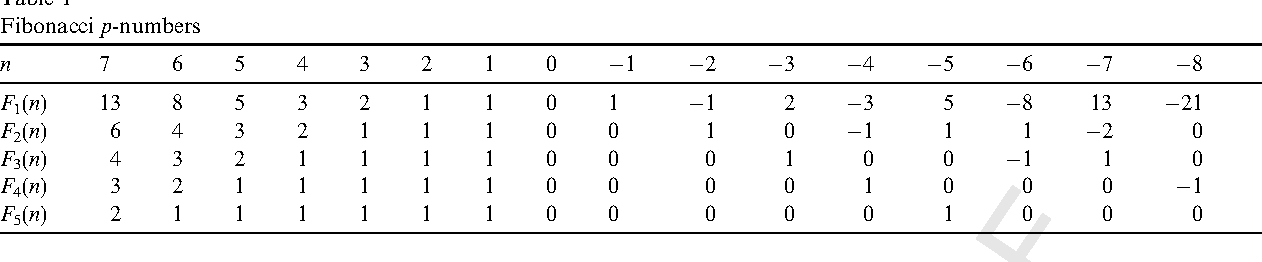•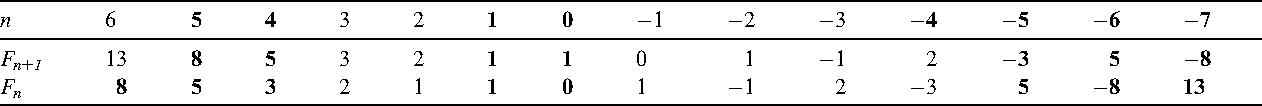Is this relevant?
2003
2003
In this paper, we have constituted 3-step Fibonacci sequences by the three generating elements of a group of exponent p (p is… Expand
Is this relevant?
1998
1998
The study of Fibonacci sequences in groups began with the earlier work of Wall , where the ordinary Fibonacci sequences in… Expand
Is this relevant?